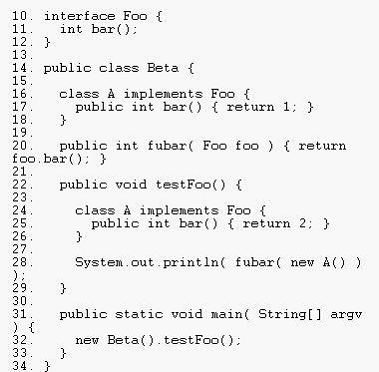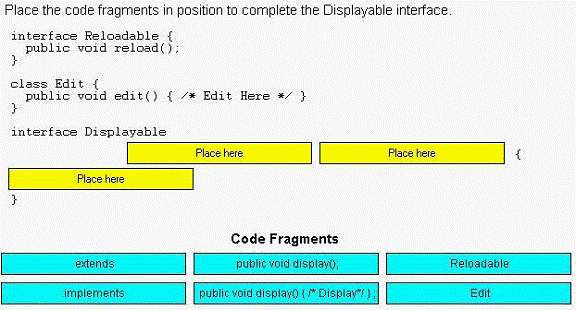### Language Fundamentals SCJP / OCJP

You are here : HomeCore Java Tutorials /  SCJP / OCJP dumps

Question: 1 Given
10. class Foo {
11. static void alpha() { /* more code here */ }
12. void beta() { /* more code here */ }
13. }
Which two statements are true? (Choose two.)
A. Foo.beta() is a valid invocation of beta().
B. Foo.alpha() is a valid invocation of alpha().
C. Method beta() can directly call method alpha().
D. Method alpha() can directly call method beta().

Question
Question: 2 Given
12. public class Yippee2 {
13.
14. static public void main(String [] yahoo) {
15. for(int x = 1; x < yahoo.length; x++) {
16. System.out.print(yahoo[x] + " ");
17. }
18. }
19. }
and the command line invocation:
java Yippee2 a b c
What is the result?
A. a b
B. b c
C. a b c
D. Compilation fails.
E. An exception is thrown at runtime.

Question: 3 Given:
15. public class Yippee {
16. public static void main(String [] args) {
17. for(int x = 1; x < args.length; x++) {
18. System.out.print(args[x] + " ");
19. }
20. }
21. }
and two separate command line invocations:
java Yippee
java Yippee 1 2 3 4
What is the result?
A.

No output is produced.
1 2 3
B. No output is produced.
2 3 4
C. No output is produced.
1 2 3 4
D. An exception is thrown at runtime.
1 2 3
E. An exception is thrown at runtime.
2 3 4
F. An exception is thrown at runtime.
1 2 3 4

Q: 4 Given a class Repetition:
1. package utils;
2.
3. public class Repetition {
4. public static String twice(String s) { return s + s; }
5. }
and given another class Demo:
1. // insert code here
2.
3. public class Demo {
4. public static void main(String[] args) {
5. System.out.println(twice("pizza"));
6. }
7. }
Which code should be inserted at line 1 of Demo.java to compile and run Demo to print "pizzapizza"?
A. import utils.*;
B. static import utils.*;
C. import utils.Repetition.*;
D. static import utils.Repetition.*;
E. import utils.Repetition.twice();
F. import static utils.Repetition.twice;
G. static import utils.Repetition.twice;

Q: 5 A JavaBeans component has the following field:
11. private boolean enabled;
Which two pairs of method declarations follow the JavaBeans standard for accessing this field? (Choose two.)
A. public void setEnabled( boolean enabled )
public boolean getEnabled()
B. public void setEnabled( boolean enabled )
public void isEnabled()
C. public void setEnabled( boolean enabled )
public boolean isEnabled()
D. public boolean setEnabled( boolean enabled )
public boolean getEnabled()

Q: 6
Given classes defined in two different files:
1. package util;
2. public class BitUtils {
3. public static void process(byte[]) { /* more code here */ }
4. }
1. package app;
2. public class SomeApp {
3. public static void main(String[] args) {
4. byte[] bytes = new byte;
5. // insert code here
6. }
7. }
What is required at line 5 in class SomeApp to use the process method of BitUtils?
A. process(bytes);
B. BitUtils.process(bytes);
C. util.BitUtils.process(bytes);
D. SomeApp cannot use methods in BitUtils.
E. import util.BitUtils.*; process(bytes);

Q: 7 Given:
enum Example { ONE, TWO, THREE }
Which statement is true?
A. The expressions (ONE == ONE) and ONE.equals(ONE) are both guaranteed to be true.
B. The expression (ONE < TWO) is guaranteed to be true and ONE.compareTo(TWO) is guaranteed to be less than one.
C. The Example values cannot be used in a raw java.util.HashMap; instead, the programmer must use a java.util.EnumMap.
D. The Example values can be used in a java.util.SortedSet, but the set will NOT be sorted because enumerated types do NOT implement java.lang.Comparable.

Q: 8 Given:
11. public abstract class Shape {
12. private int x;
13. private int y;
14. public abstract void draw();
15. public void setAnchor(int x, int y) {
16. this.x = x;
17. this.y = y;
18. }
19. }
Which two classes use the Shape class correctly? (Choose two.)
A. public class Circle implements Shape {
}
B. public abstract class Circle extends Shape {
}
C. public class Circle extends Shape {
public void draw();
}
D. public abstract class Circle implements Shape {
public void draw();
}
E. public class Circle extends Shape {
public void draw() {/* code here */}
F. public abstract class Circle implements Shape {
public void draw() { /* code here */ }

Also read : SCJP / OCJP dumps

Q: 09 Given:
10. class Nav{
11. public enum Direction { NORTH, SOUTH, EAST, WEST }
12. }
13. public class Sprite{
14. // insert code here
15. }
Which code, inserted at line 14, allows the Sprite class to compile?
A. Direction d = NORTH;
B. Nav.Direction d = NORTH;
C. Direction d = Direction.NORTH;
D. Nav.Direction d = Nav.Direction.NORTH;

Q: 10 Click the Exhibit button.
Which three statements are true? (Choose three.)A. Compilation fails.
B. The code compiles and the output is 2.
C. If lines 16, 17 and 18 were removed, compilation would fail.
D. If lines 24, 25 and 26 were removed, compilation would fail.
E. If lines 16, 17 and 18 were removed, the code would compile and
the output would be 2.
F. If lines 24, 25 and 26 were removed, the code would compile and
the output would be 1.

Q: 11 Click the Task button.Solution:
}
class Edit{
public void edit(){/* Edit Here*/}
}
interface Displayable
public void display();

Q:12 Given:
35. String #name = "Jane Doe";
36. int \$age = 24;
37. Double _height = 123.5;
38. double ~temp = 37.5;
Which two statements are true? (Choose two.)
A. Line 35 will not compile.
B. Line 36 will not compile.
C. Line 37 will not compile.
D. Line 38 will not compile.

Q: 13 Given:
55. int [] x = {1, 2, 3, 4, 5};
56. int y[] = x;
57. System.out.println(y);
Which statement is true?
A. Line 57 will print the value 2.
B. Line 57 will print the value 3.
C. Compilation will fail because of an error in line 55.
D. Compilation will fail because of an error in line 56.

Q: 14
A programmer needs to create a logging method that can accept an
arbitrary number of arguments. For example, it may be called in these ways:
logIt("log message1");
logIt("log message2","log message3");
logIt("log message4","log message5","log message6");
Which declaration satisfies this requirement?
A. public void logIt(String * msgs)
B. public void logIt(String [] msgs)
C. public void logIt(String... msgs)
D. public void logIt(String msg1, String msg2, String msg3)

Q: 15
Which two code fragments correctly create and initialize a static array of int
elements? (Choose two.)
A. static final int[] a = { 100,200 };
B. static final int[] a;
static { a=new int; a=100; a=200; }
C. static final int[] a = new int{ 100,200 };
D. static final int[] a;
static void init() { a = new int; a=100; a=200; }

Q: 16 Given:
11. public static void main(String[] args) {
12. String str = "null";
13. if (str == null) {
14. System.out.println("null");
15. } else (str.length() == 0) {
16. System.out.println("zero");
17. } else {
18. System.out.println("some");
19. }
20. }
What is the result?
A. null
B. zero
C. some
D. Compilation fails.
E. An exception is thrown at runtime.

Q: 17 Click the Exhibit button.
Given:
34. Test t = new Test();
35. t.method(5);
What is the output from line 5 of the Test class?
1. public class Test {
2. int x= 12;
3. public void method(int x) {
4. x+=x;
5. System.out.println(x);
6. }
7. }

A. 5
B. 10
C. 12
D. 17

Q: 18 Given
11. public interface Status {
12. /* insert code here */ int MY_VALUE = 10;
13. }
Which three are valid on line 12? (Choose three.)
A. final
B. static
C. native
D. public
E. private
F. abstract
G. protected

Question: 19
A programmer is designing a class to encapsulate the information
about an inventory item. A JavaBeans component is needed to
do this. The Inventoryltem class has private instance variables to store
the item information:
10. private int itemId;
11. private String name;
12. private String description;
Which method signature follows the JavaBeans naming standards for
modifying the itemld instance variable?
A. itemID(int itemId)
B. update(int itemId)
C. setItemId(int itemId)
D. mutateItemId(int itemId)
E. updateItemID(int itemId)

Question:20
Given a file GrizzlyBear.java:
1. package animals.mammals;
2.
3. public class GrizzlyBear extends Bear {
4. void hunt() {
5. Salmon s = findSalmon();
6. s.consume();
7. }
8. }
and another file, Salmon.java:
1. package animals.fish;
2.
3. public class Salmon extends Fish {
4. void consume() { /* do stuff */ }
5. }
Assume both classes are defined in the correct directories for theft
packages, and that the Mammal class correctly defines the
findSalmon() method. Which two changes allow this code to compile
correctly? (Choose two.)
A. add public to the start of line 4 in Salmon.java
B. add public to the start of line 4 in GrizzlyBear.java
C. add import animals.mammals.*; at line 2 in Salmon.java
D. add import animals.fish.*; at line 2 in GrizzlyBear.java
E. add import animals.fish.Salmon.*; at line 2 in GrizzlyBear.java
F. add import animals.mammals.GrizzlyBear.*;at line 2 in Salmon.java

21. Which are valid declarations? (Choose all that apply.)
A. int \$x;
Bint 123;
C. int _123;
D. int #dim;
E. int %percent;
F. int *divide;
G. int central_sales_region_Summer_2005_gross_sales;
-> A, C, and are legal identifiers.
D, E, and are incorrect because identifiers must start with \$, _, or a letter.

22. Which method names follow the JavaBeans standard? (Choose all that apply.)
BgetCust
C. deleteRep
E. putDimensions
-> and use the valid prefixes 'get' and 'is'.
->A, C, and are incorrect because 'add', 'delete' and 'put' are not standard JavaBeans name prefixes.

23. Given:
1. class Voop {
2. public static void main(String[] args) {
3. doStuff(1);
4. doStuff(1,2);
5. }
6. // insert code here
7. }
Which, inserted independently at line 6, will compile? (Choose all that apply.)
A. static void doStuff(int... doArgs) { }
Bstatic void doStuff(int[] doArgs) { }
C. static void doStuff(int doArgs...) { }
D. static void doStuff(int... doArgs, int y) { }
E. static void doStuff(int x, int... doArgs) { }
-> and E use valid var-args syntax.
-> and are invalid var-arg syntax, and is invalid because the var-arg must be the last of a method's arguments. (Objective 1.4)

24. Which are legal declarations? (Choose all that apply.)
A. short x [];
Bshort [] y;
C. short x2;
D. short z2 ;
E. short [] z [] [];
F. short [] y2 = ;